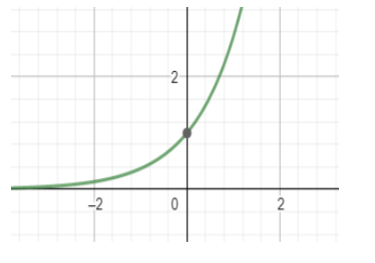Courses
Courses for Kids
Free study material
Free LIVE classes
MoreLIVE
Join Vedantu’s FREE Mastercalss

# Find the range of function f(x) where f(x) = ${{e}^{x}}$ in [0, 1].Verified
363.3k+ views
Hint: We have to first check the continuity of the function in the given interval. Then we have to find the limits of the function at its extreme intervals.

So here we are given a function ${{e}^{x}}$ in [0, 1] and we have to find its range.
First we need to find if this function is continuous in [0, 1].
f (x) = ${{e}^{x}}$
f (0) = ${{e}^{0}}$
= 1
f (1) =${{e}^{1}}$
= e
As the limits exist in the interval and there are no corner points where the function becomes discontinuous, we can say that the function is continuous in the interval [0, 1].
Here the function f(x) = ${{e}^{x}}$ is increasing in nature, which means f(x+1)>f(x).
So f(1) > f(0)
f (0) = ${{e}^{0}}$
= 1
f (1) =${{e}^{1}}$
= eThis is the graph of ${{e}^{x}}$. We can see that the function tends to 0 when x tends to -∞. And when x tends to ∞ then the function ${{e}^{x}}$ also tends to infinity. It intersects the y axis at the point (0,1) which means when the value of x = 0 , then the value of the function is 1.
We can see this is an increasing function and it is continuous in the interval [0, 1].
Therefore the range of the function f(x) = ${{e}^{x}}$ is [0, 1]
Note: We must remember the graphs of ${{e}^{x}}$ and ${{e}^{-x}}$ as they are very much important for boards as well as competitive exams. Where ${{e}^{x}}$ is always increasing in nature and on the other hand ${{e}^{-x}}$ is always decreasing in nature. They are also called exponential graphs, which are used to model populations.
Last updated date: 25th Sep 2023
Total views: 363.3k
Views today: 5.63k